# The Nature Of Light | HSC Physics Study Guide Part 3

The Year 12 Physics Nature of Light module is the first introduction to modern physics: quantum mechanics and special relativity. These concepts are challenging and non-intuitive and students very often struggle with them. Students must develop a clear understanding of these and learn how to apply them to obtain full marks in exam questions. In this third part of the HSC Physics study guide, we present key ideas from this topic in a distilled, logical way using flowcharts.

Matrix students are encouraged to organise complex ideas in ways that are easy to understand and visualise, and are taught how to use such techniques to answer longer exam questions.

## 1. The Wave Nature of Light

The earliest debate as to the nature of light that is studied in this topic is between Huygens’ wave model and Newton’s corpuscular (particle) model.

While both matched the observed properties of light at the time, later experiments proved Huygens correct: light was a wave. This is outlined in the flowchart below: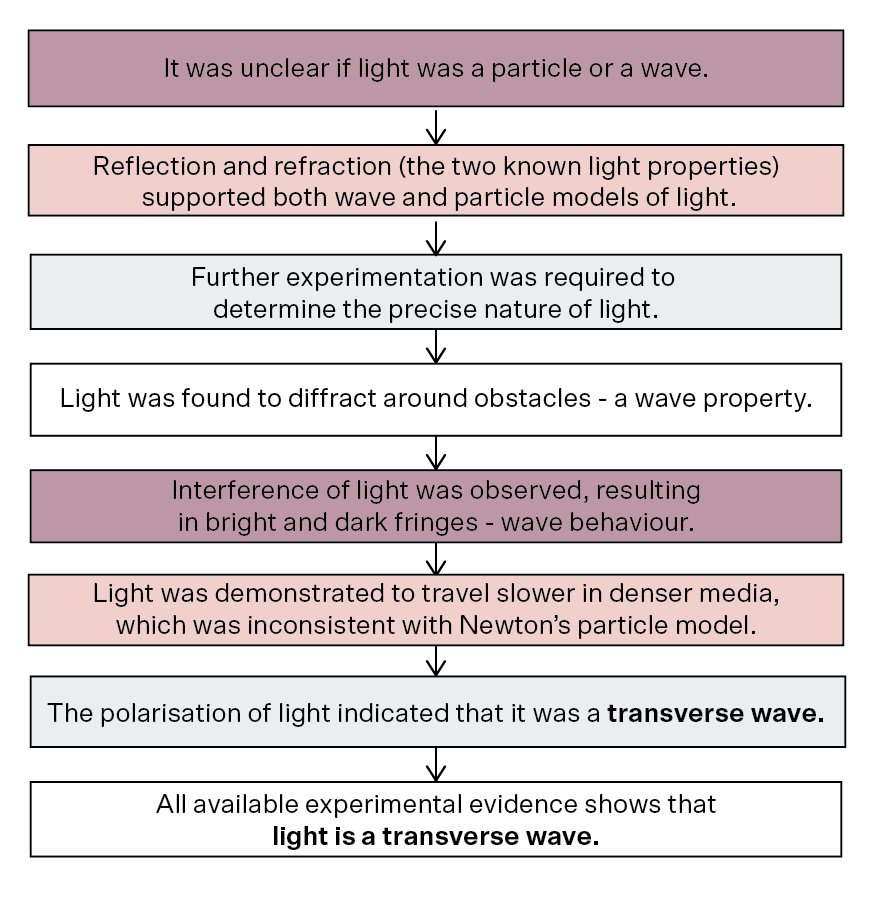## 2. What Type of Wave is Light?

Following the conclusion that light was a transverse wave, the question remained: what type of wave was it?

The two scientist most responsible for the answer were James Maxwell and Heinrich Hertz, who concluded that light is an electromagnetic wave.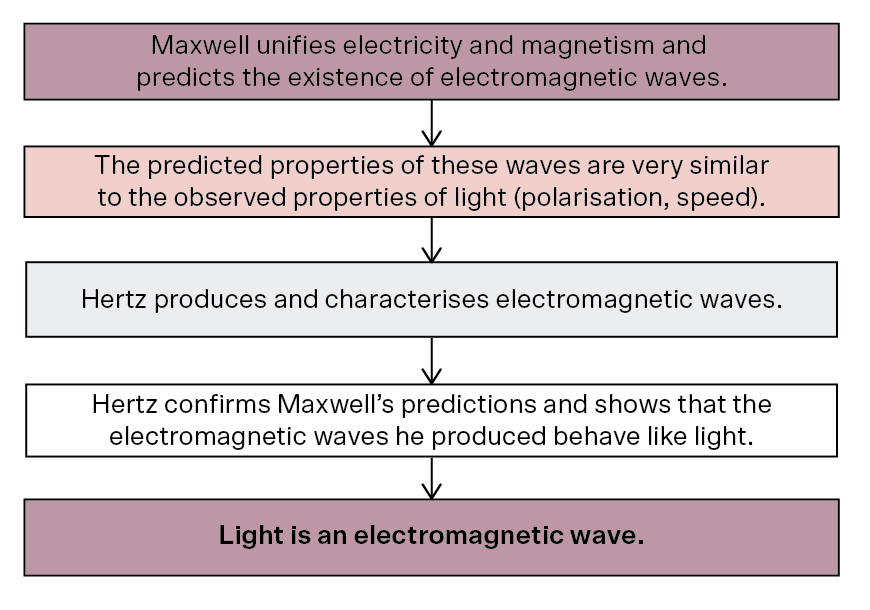## 3. The Quantisation of Light

The concept of the quantisation of light and the photon model are the cumulative achievements of Max Planck and Albert Einstein.

Planck suggested the notion of quantisation to better predict the emission spectra of black bodies, which was poorly explained by classical physics. Einstein expanded this idea to light, developing the photon model: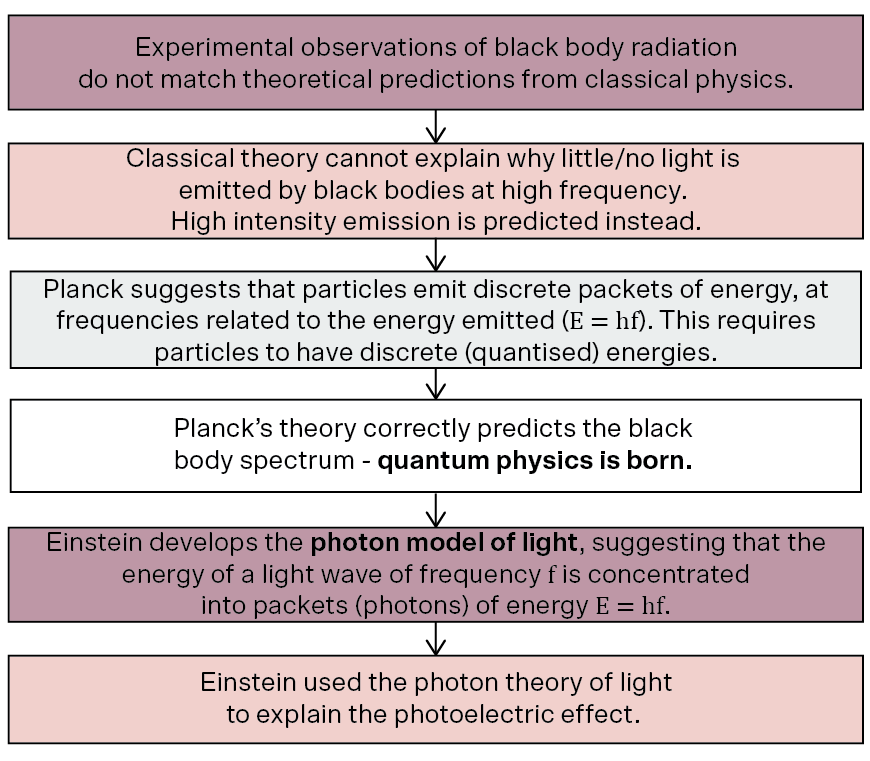## 4. The Photoelectric Effect

The phenomenon that led Einstein to develop the photon model of light was the photoelectric effect, where light above a certain frequency caused electrons to be ejected from a metal.

Einstein’s explanation is outlined below: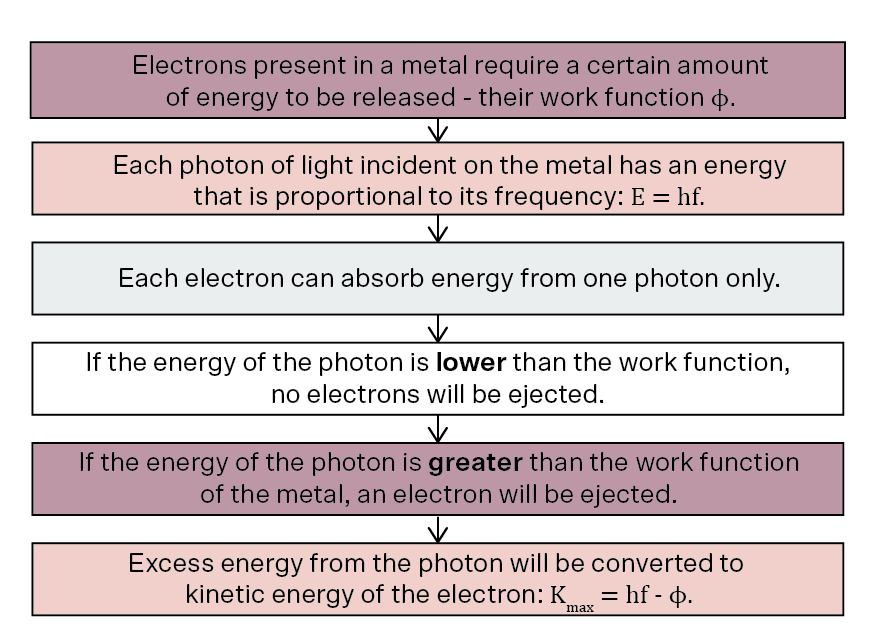## 5. Special Relativity – Time Dilation and Length Contraction

One of the more confusing aspects of light is its relation to the nature of observations as outlined in Einstein’s Special Theory of Relativity.

In this, Einstein adopts two postulates – that all inertial reference frames are equivalent, and that all observers measure the same speed of light regardless of their motion or the motion of the source – and uses them to make bold predictions about contradictory observations of space and time for observers in different reference frames. This is summarised below: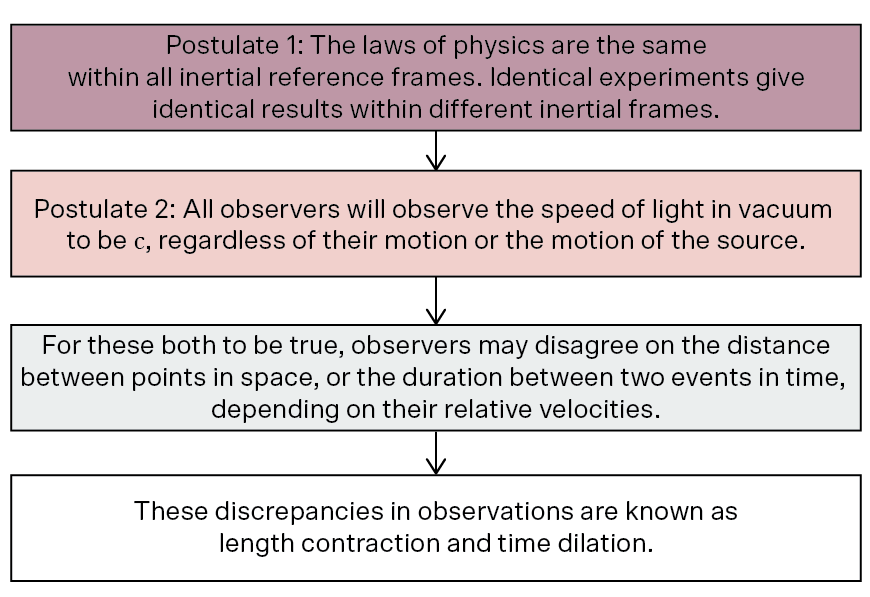## 6. Special Relativity – Mass Energy Equivalence

One of the more famous outcomes of Special Relativity is the principle of mass-energy equivalence and the corresponding equation E = mc2.

This arises from the relativistic momentum and mass dilation: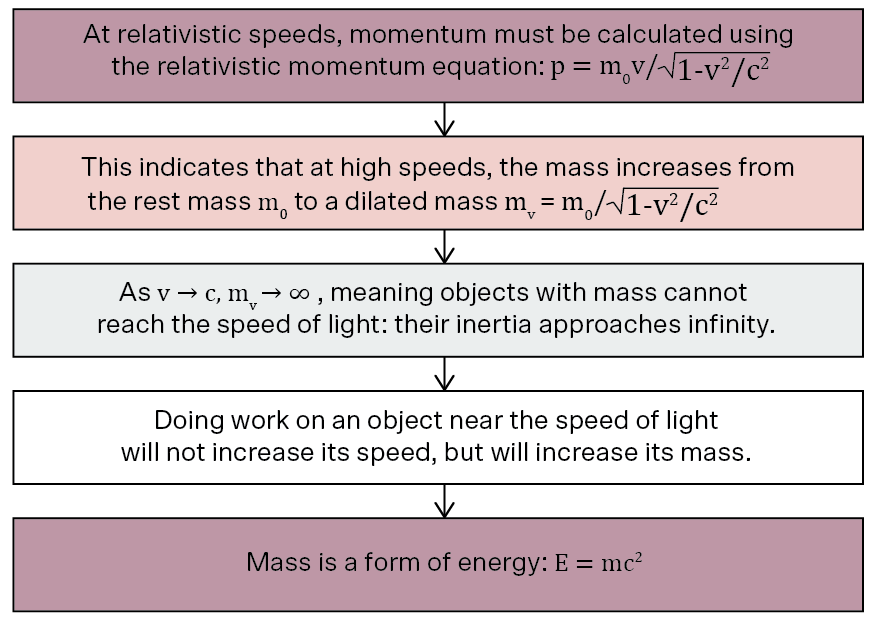#### Related Posts

Platform Slot Gacor CMS Checker